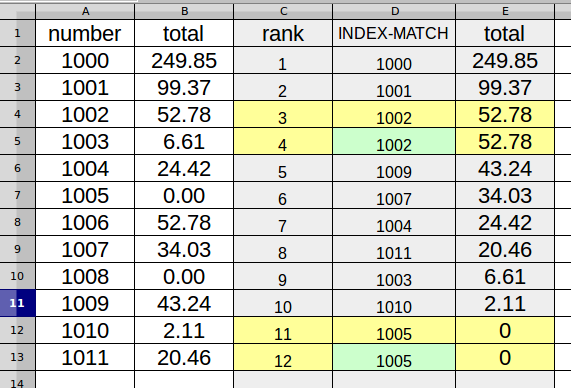# Formula to return all corresponding values from an array

Hello All, I stumbled upon a problem with VLOOKUP that it only returns first value that meets the search criterion, and so does INDEX-MATCH formula. But in my table there are repeating values so my task is to include them all (in, say, ascending order). Here is my table:Where A and B columns contain original data, C is rank, E is filled with =LARGE(\$B\$2:\$B\$138, C2) to show which B cell is the largest, the second largest and so on. I want D column to return the corresponding number from A column but using =INDEX(\$A\$2:\$A\$138, MATCH(E2, \$B\$2:\$B\$138, 0)) I found that only first match for the criteria is returned. I highlighted wrong pairs of rows with yellow so you can see that the first row of yellow rows is correct while the second returns the first value again instead of finding the next cell containing the criteria (1006 and 1008 respectively). I also highlighted wrong second value with green to make it more prominent.

I suppose column D should contain an array formula instead of INDEX-MATCH but I didn’t succeed in creating one. Please help. Thank you!

Of course MATCH() returns the same position when asked twice for the same value…

I’d simply select the data in columns A and B and sort all on the total B column, menu Data → Sort…

Thank you but sorting is not an option. I need a formula.